# Sinus

Determine the smallest integer p for which the equation 4 sin x = p has no solution.

p =  5

### Step-by-step explanation: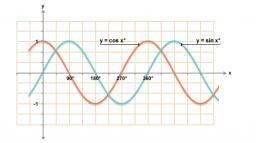Did you find an error or inaccuracy? Feel free to write us. Thank you!Tips to related online calculators
Do you have a linear equation or system of equations and looking for its solution? Or do you have a quadratic equation?
Most natural application of trigonometry and trigonometric functions is a calculation of the triangles. Common and less common calculations of different types of triangles offers our triangle calculator. Word trigonometry comes from Greek and literally means triangle calculation.

## Related math problems and questions:

• TrigonometryIs true equality? sin(x +13 π)= sin(x)
• SinesIn ▵ ABC, if sin(α)=0.5 and sin(β)=0.6 calculate sin(γ)
• DivisibilityDetermine the smallest integer which divided 11 gives remainder 4 when divided 15 gives remainder 10 and when divided by 19 gives remainder 16.
• CotangentIf the angle α is acute, and cotan α = 1/3. Determine the value of sin α, cos α, tg α.
• Trigonometric functionsIn the right triangle is: tg α= frac(2) 1 Find the value of s and c: sin α= (s)/(√ 5) cos α= (c)/(√ 5)
• TrigonometryIf you know that cos(γ) = sin (806°), what is the angle γ?
• Q-Exam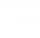If tg α = 9.6, Calculating sin α, cos α, cotg α .
• Sin cos tan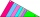In triangle ABC, right-angled at B. Sides/AB/=7cm, /BC/=5cm, /AC/=8.6cm. Find to two decimal places. A. Sine C B. Cosine C C. Tangent C.
• Z9-I-4Kate thought a five-digit integer. She wrote the sum of this number and its half at the first line to the workbook. On the second line wrote a total of this number and its one fifth. On the third row, she wrote a sum of this number and its one nines. Fina
• Diofant equationIn the set of integers (Z) solve the equation: 212x +316y =0 Write result with integer parameter t in Z (parameter t = ...-2,-1,0,1,2,3... if equation has infinitely many solutions)
• RightDetermine angles of the right triangle with the hypotenuse c and legs a, b, if: 3a +4b = 4.9c
• Angle in RTDetermine the size of the smallest internal angle of a right triangle whose sides constitutes sizes consecutive members of arithmetic progressions.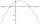It is given a quadratic function y = -4x2+5x+c with unknown coefficient c. Determine the smallest integer c for which the graph of f intersects the x-axis at two different points.250x + 120y = 5640Is equation 70x +52y = 34 solvable on the set of integers Z?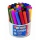600 pencils we want to be divided into three groups. The biggest groups have ten pens more than the smallest. How many ways can this be done?The sum of seven consecutive odd natural numbers is 119. Determine the smallest of them.